# PSAT Math : How to multiply even numbers

## Example Questions

### Example Question #52 : Integers

If x is an even integer and y is an odd integer. Which of these expressions represents an odd integer?

I. xy

II. x-y

III. 3x+2y

II and III only

I and III only

II only

I and II only

I, II, and III only

II only

Explanation:

I)xy is Even*Odd is Even. II) x-y is Even+/-Odd is Odd. III) 3x is Odd*Even =Even, 2y is Even*Odd=Even, Even + Even = Even. Therefore only II is Odd.

### Example Question #1 : How To Multiply Even Numbers

If x is an even number, y is an odd number, and z is an even number, which of the following will always give an even number?

I. xyz

II. 2x+3y

III. z– y

I only

II only

II and III only

I, II and III

I and II only

I only

Explanation:

I. xyz = even * odd * even = even

II. 2x + 3y = even*even + odd*odd = even + odd = odd

III. z– y = even * even – odd = even – odd = odd

Therefore only I will give an even number.

### Example Question #51 : Integers

If x and are integers and at least one of them is even, which of the following MUST be true?

x + y is odd

xy is odd

Nothing can be determined based on the given information

xis even

xy is even

xy is even

Explanation:

Since we are only told that "at least" one of the numbers is even, we could have one even and one odd integer OR we could have two even integers.

Even plus odd is odd, but even plus even is even, so xy could be either even or odd.

Even times odd is even, and even times even is even, so xy must be even.

### Example Question #1 : How To Multiply Even Numbers

Let a and b be positive integers such that ab2 is an even number. Which of the following must be true?

I. ais even

II. a2b is even

III. ab is even

I and II only

II and III only

I only

I, II, and III

II only

II and III only

Explanation:

In order to solve this problem, it will help us to find all of the possible scenarios of a, b, a2, and b2. We need to make use of the following rules:

1. The product of two even numbers is an even number.

2. The product of two odd numbers is an odd number.

3. The product of an even and an odd number is an even number.

The information that we are given is that ab2 is an even number. Let's think of ab2 as the product of two integers: a and b2.

In order for the product of a and b2 to be even, at least one of them must be even, according to the rules that we discussed above. Thus, the following scenarios are possible:

Scenario 1: a is even and b2 is even

Scenario 2: a is even and b2 is odd

Scenario 3: a is odd and b2 is even

Next, let's consider what possible values are possible for b. If b2 is even, then this means b must be even, because the product of two even numbers is even. If b were odd, then we would have the product of two odd numbers, which would mean that b2 would be odd. Thus, if b2 is even, then b must be even, and if b2 is odd, then b must be odd. Let's add this information to the possible scenarios:

Scenario 1: a is even, b2 is even, and b is even

Scenario 2: a is even, b2 is odd, and b is odd

Scenario 3: a is odd, b2 is even, and b is even

Lastly, let's see what is possible for a2. If a is even, then amust be even, and if a is odd, then a2 must also be odd. We can add this information to the three possible scenarios:

Scenario 1: a is even, b2 is even, and b is even, and a2 is even

Scenario 2: a is even, b2 is odd, and b is odd, and a2 is even

Scenario 3: a is odd, b2 is even, and b is even, and a2 is odd

Now, we can use this information to examine choices I, II, and III.

Choice I asks us to determine if a2 must be even. If we look at the third scenario, in which a is odd, we see that a2 would also have to be odd. Thus it is possible for a2 to be odd.

Next, we can analyze a2b. In the first scenario, we see that a2 is even and b is even. This means that a2b would be even. In the second scenario, we see that a2 is even, and b is odd, which would still mean that a2b is even. And in the third scenario, a2 is odd and b is even, which also means that a2b would be even. In short, a2b is even in each of the possible scenarios, so it must always be even. Thus, choice II must be true.

We can now look at ab. In scenario 1, a is even and b is even, which means that ab would also be even. In scenario 2, a is even and b is odd, which means that ab is even again. And in scenario 3, a is odd and b is even, which again means that ab is even. Therefore, ab must be even, and choice III must be true.

The answer is II and III only.

### Example Question #1 : How To Multiply Even Numbers

Let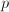equal the product of two numbers.  If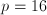, then the two numbers COULD be which of the following?

8 and 8

0 and 16

2 and 8

20 and 4

32 and 2

2 and 8

Explanation:

The word "product" refers to the answer of a multiplication problem.  Since 2 times 8 equals 16, it is a valid pair of numbers.

### Example Question #21 : Even / Odd Numbers

If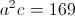andis an odd integer, which of the following couldbe divisible by?Explanation:

Ifis an odd integer then we can plug 1 intoand solve foryielding 13. 13 is prime, meaning it is only divisible by 1 and itself.

### Example Question #271 : Arithmetic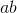is even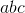is even

Therefore, which of the following must be true about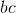?

It must be even.

It must be odd.

It could be either even or odd.

It could be either even or odd.

Explanation:

Recall that when you multiply by an even number, you get an even product.

Therefore, we know the following from the first statement:is even oris even or bothandare even.

For the second, we know this:

Sinceis even, therefore,can be either even or odd. (Regardless of what it is, we can get an even value for.)

Based on all this data, we can tell nothing necessarily about. Ifis even, thenis even, even ifis odd. However, ifis odd whileis even, thenwill be even.

### Example Question #1 : How To Multiply Even Numbers

In a group of philosophers,are followers of Durandus. Twice that number are followers of Ockham. Four times the number of followers of Ockham are followers of Aquinas. One sixth of the number of followers of Aquinas are followers of Scotus. How many total philosophers are in the group?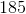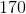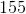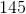Explanation:

In a group of philosophers,are followers of Durandus. Twice that number are followers of Ockham. Four times the number of followers of Ockham are followers of Aquinas. One sixth of the number of followers of Aquinas are followers of Scotus. How many total philosophers are in the group?

To start, let's calculate the total philosophers:

Ockham:* <Number following Durandus>, or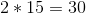Aquinas:* <Number following Ockham>, or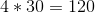Scotus:divided by, orTherefore, the total number is: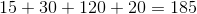### Example Question #1 : How To Multiply Even Numbers

If n is an integer that is not equal to 0, which of the following must be greater than or equal to n?

I. 7n

II. n + 5

III. n2

II and III only

I and II only

I and III only

II only

I, II, and III

II and III only

Explanation:

I is not always true because a negative number multiplied by 7 will give a number that is more negative than the original. II is true because adding 5 to any number will increase the value. III is true because squaring any number will increase the magnitude of the value, and squaring a negative number will make it positive.

### Example Question #1 : How To Multiply Even Numbers

Which of the following integers has an even integer value for all positive integers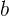and?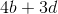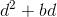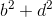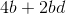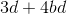There are certain patterns that can be used to predict whether the product or sum of numbers will be odd or even. The sum of two odd numbers is always even, as is the sum of two even numbers. The sum of an odd number and an even number is always odd. In multiplication the product of two odd numbers is always odd. While the product of even numbers, as well as the product of odd numbers multiplied by even numbers is always even. So for this problem we need to find scenarios where the only possibile answers are even.can only result in even numbers no matter what positive integers are used forand, because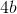must can only result in even products; the same can be said for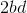. The rules provide that the sum of two even numbers is even, sois the answer.